Search by Topic

Resources tagged with Graph sketching similar to Exponential Trend:

Filter by: Content type:
Age range:
Challenge level:

There are 27 results

Broad Topics > Functions and Graphs > Graph sketchingQuartics

Age 16 to 18 Challenge Level:

Investigate the graphs of y = [1 + (x - t)^2][1 + (x + t^)2] as the parameter t varies.Curve Match

Age 16 to 18 Challenge Level:

Which curve is which, and how would you plan a route to pass between them?What's That Graph?

Age 14 to 16 Challenge Level:

Can you work out which processes are represented by the graphs?A Close Match

Age 16 to 18 Challenge Level:

Can you massage the parameters of these curves to make them match as closely as possible?Curve Fitter

Age 16 to 18 Challenge Level:

Can you fit a cubic equation to this graph?Sine Problem

Age 16 to 18 Challenge Level:

In this 'mesh' of sine graphs, one of the graphs is the graph of the sine function. Find the equations of the other graphs to reproduce the pattern.Pitchfork

Age 16 to 18 Challenge Level:

Plot the graph of x^y = y^x in the first quadrant and explain its properties.Bio Graphs

Age 14 to 16 Challenge Level:

What biological growth processes can you fit to these graphs?Guess the Function

Age 16 to 18 Challenge Level:

This task depends on learners sharing reasoning, listening to opinions, reflecting and pulling ideas together.Whose Line Graph Is it Anyway?

Age 16 to 18 Challenge Level:

Which line graph, equations and physical processes go together?Folium of Descartes

Age 16 to 18 Challenge Level:

Investigate the family of graphs given by the equation x^3+y^3=3axy for different values of the constant a.Witch of Agnesi

Age 16 to 18 Challenge Level:

Sketch the members of the family of graphs given by y = a^3/(x^2+a^2) for a=1, 2 and 3.Slide

Age 16 to 18 Challenge Level:

This function involves absolute values. To find the slope on the slide use different equations to define the function in different parts of its domain.Curvy Catalogue

Age 16 to 18 Challenge Level:

Make a catalogue of curves with various properties.Area L

Age 16 to 18 Challenge Level:

By sketching a graph of a continuous increasing function, can you prove a useful result about integrals?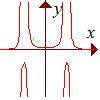Rational Request

Age 16 to 18 Challenge Level:

Can you make a curve to match my friend's requirements?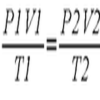Ideal Axes

Age 16 to 18 Challenge Level:

Explore how can changing the axes for a plot of an equation can lead to different shaped graphs emerging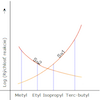Reaction Types

Age 16 to 18 Challenge Level:

Explore the rates of growth of the sorts of simple polynomials often used in mathematical modelling.Scientific Curves

Age 16 to 18 Challenge Level:

Can you sketch these difficult curves, which have uses in mathematical modelling?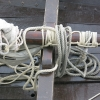Tangled Trig Graphs

Age 16 to 18 Challenge Level:

Can you work out the equations of the trig graphs I used to make my pattern?Back Fitter

Age 14 to 16 Challenge Level:

10 graphs of experimental data are given. Can you use a spreadsheet to find algebraic graphs which match them closely, and thus discover the formulae most likely to govern the underlying processes?How Does Your Function Grow?

Age 16 to 18 Challenge Level:

Compares the size of functions f(n) for large values of n.Cocked Hat

Age 16 to 18 Challenge Level:

Sketch the graphs for this implicitly defined family of functions.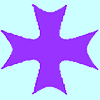Maltese Cross

Age 16 to 18 Challenge Level:

Sketch the graph of $xy(x^2 - y^2) = x^2 + y^2$ consisting of four curves and a single point at the origin. Convert to polar form. Describe the symmetries of the graph.Squareness

Age 16 to 18 Challenge Level:

The family of graphs of x^n + y^n =1 (for even n) includes the circle. Why do the graphs look more and more square as n increases?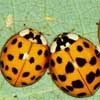Spot the Difference

Age 16 to 18 Short Challenge Level:

If you plot these graphs they may look the same, but are they?Polar Flower

Age 16 to 18 Challenge Level:

This polar equation is a quadratic. Plot the graph given by each factor to draw the flower.